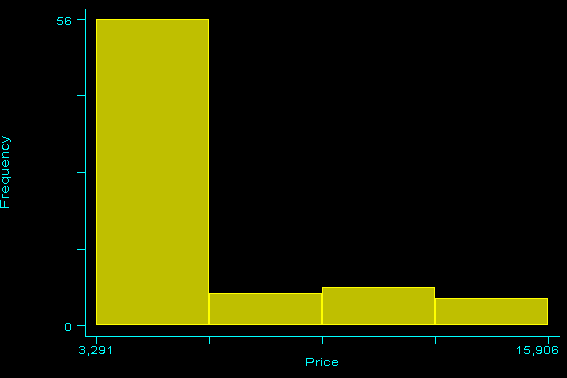»  Home »  Resources & support »  FAQs »  Frequency plots
Note: This FAQ is relevant for users of releases prior to Stata 8.

## How do I make a frequency plot using Stata?

 Title Frequency plots Author Jeremy B. Wernow, StataCorp

Frequency plots can be made in Stata using the hist command with the freq option.

  . hist mpg, freq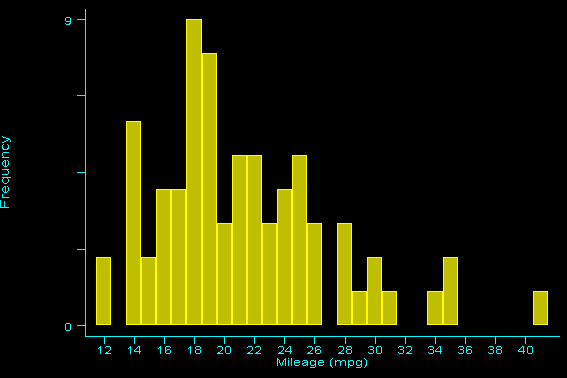. hist rep78, freq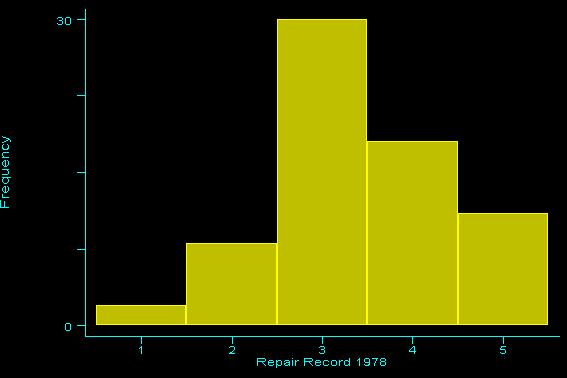However, if the variable you are graphing takes on noninteger values, this command will not work.

  . describe headroom

storage  display     value
variable name   type   format      label      variable label
------------------------------------------------------------------------

(in.) |      Freq.     Percent        Cum.
------------+-----------------------------------
1.5 |          4        5.41        5.41
2.0 |         13       17.57       22.97
2.5 |         14       18.92       41.89
3.0 |         13       17.57       59.46
3.5 |         15       20.27       79.73
4.0 |         10       13.51       93.24
4.5 |          4        5.41       98.65
5.0 |          1        1.35      100.00
------------+-----------------------------------
Total |         74      100.00

headroom must take on integer values
r(198);


An alternate solution would be to create a group variable, and then use the graph command with the freq, hist, and bin() options.

  . egen y = group(headroom)

. list y headroom in 1/10

1.         3     2.5
2.         4     3.0
3.         4     3.0
4.         7     4.5
5.         6     4.0
6.         6     4.0
7.         4     3.0
8.         2     2.0
9.         5     3.5
10.         5     3.5

. summarize y

Variable |     Obs        Mean   Std. Dev.       Min        Max
-------------+-----------------------------------------------------
y |      74    3.986486    1.69199          1          8

. local x = r(max) - r(min) + 1

. graph headroom, freq hist bin(x')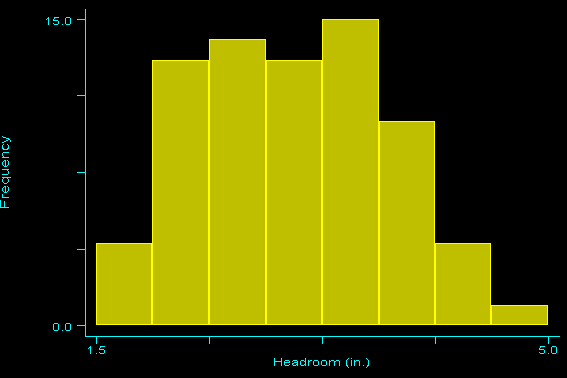The difference between the minimum and maximum values of the new group variable will provide the value needed for the bin option. The minimum and maximum values are obtained by first summarizing the group variable and then putting the saved results into a local macro. Typing this macro every time could become very tedious. An easier solution would be to create an ado-file. Here is a general example of such a file:

        -----------------------------------------freqplot.ado
program define freqplot
version 7.0
syntax varlist(max=1)
tempvar y
qui egen y' = group(varlist')
qui sum y'
local x = int(r(max)) - int(r(min)) + 1
graph varlist', histogram freq bin(x')
end


Note: If you save this file using your browser, save it as a .txt file and put double quotes around the name; i.e., enter the filename:

        "freqplot.ado"


Be sure you include the double quotes so that the file will not be saved as freqplot.ado.txt.

Suppose you wish to graph a continuous variable as opposed to a categorical variable. In this case, there is no obvious choice for the number of bins to use. If the range of the variable is relatively small, you might try the following method:

  . set obs 200
obs was 0, now 200

. gen x = uniform()*10

. summarize x

Variable |     Obs        Mean   Std. Dev.       Min        Max
-------------+-----------------------------------------------------
x |     200    4.920775   2.874816   .0572334   9.844069

. local y = int(r(max)+1) - int(r(min))

. graph x, freq hist bin(y') xlabel ylabel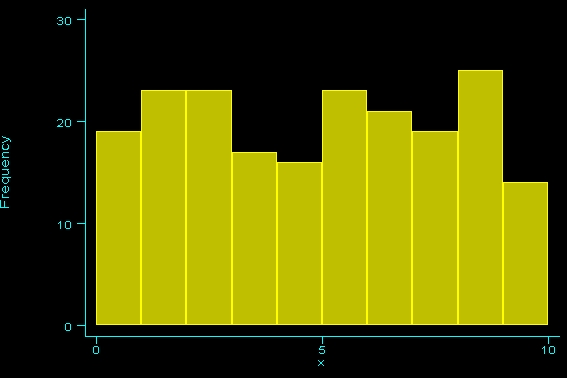Suppose, however, that the range is much larger. The simple way to approach this problem is to just declare the bin size manually.

  . graph price, freq hist bin(4)
`